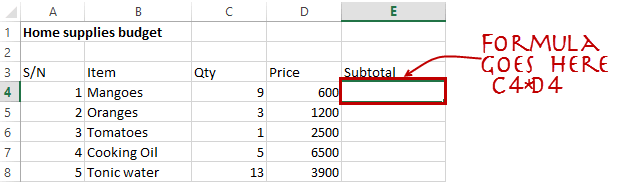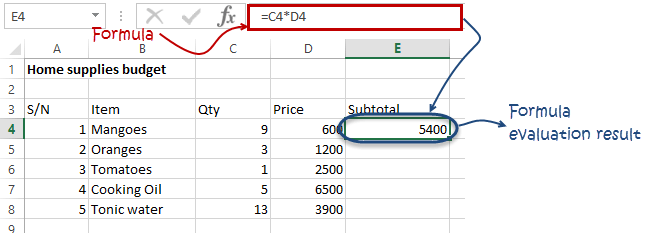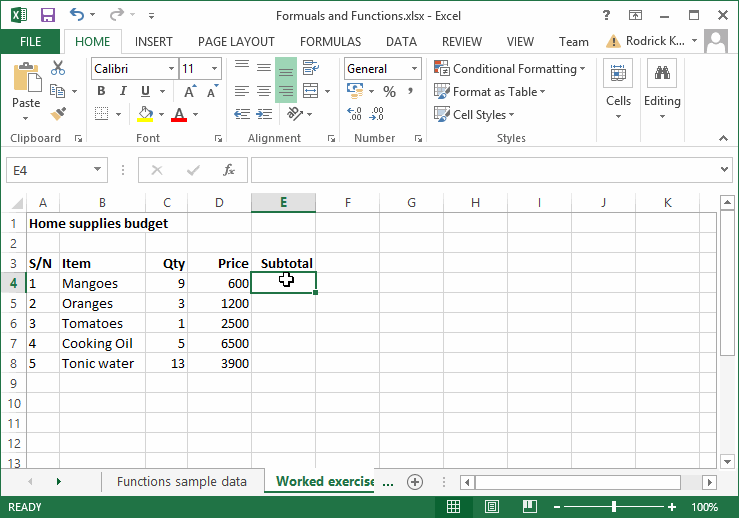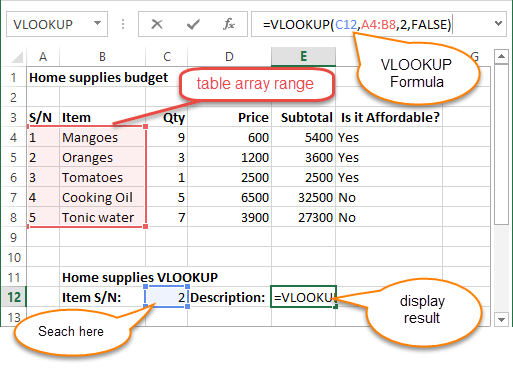## What is a formula?

It is the simplest form, a formula is an expression made up of cell addresses and arithmetic operators. Formulas can also be made up of discrete values i.e. =6*3. Excel evaluates the formula to a value. An example of a formula looks as follows.

=A2 * D2 / 2

HERE,

• “=” tells Excel that this is a formula, and it should evaluate it.
• “A2″ * D2” makes reference to cell addresses A2 and D2 then multiplies the values found in these cell addresses.
• “/” is the division arithmetic operator
• “2” is a discrete value

### Formulas practical exercise

We will work with the sample data for the home budget to calculate the subtotal.

• Create a new workbook in Excel
• Enter the data shown in the home supplies budget above.
• Your worksheet should look as follows.

——————————–

## Sample Tutorials Data For Practice

For this tutorial, we will work with the following datasets.

Home supplies budget

 S/N ITEM QTY PRICE SUBTOTAL Is it Affordable? 1 Mangoes 9 600 2 Oranges 3 1200 3 Tomatoes 1 2500 4 Cooking Oil 5 6500 5 Tonic Water 13 3900

House Building Project Schedule

 S/N ITEM START DATE END DATE DURATION (DAYS) 1 Survey land 04/02/2015 07/02/2015 2 Lay Foundation 10/02/2015 15/02/2015 3 Roofing 27/02/2015 03/03/2015 4 Painting 09/03/2015 21/03/2015

——————————————-We will now write the formula that calculates the subtotal

Set the focus to cell E4

Enter the following formula.

=C4*D4

HERE,

• “C4*D4” uses the arithmetic operator multiplication (*) to multiply the value of the cell address C4 and D4.

Press enter key

You will get the following resultThe following animated image shows you how to auto select cell address and apply the same formula to other rows.## Mistakes to avoid when working with formulas in Excel

1. Remember the rules of Brackets of Division, Multiplication, Addition, & Subtraction (BODMAS).This means expressions are brackets are evaluated first. For arithmetic operators, the division is evaluated first followed by multiplication then addition and subtraction is the last one to be evaluated. Using this rule, we can rewrite the above formula as =(A2 * D2) / 2. This will ensure that A2 and D2 are first evaluated then divided by two.
2. Formulas usually work with numeric data; you can take advantage of data validation to specify the type of data that should be accepted by a cell i.e. numbers only.
3. To ensure that you are working with the correct cell addresses referenced in the formulas, you can press F2 on the keyboard. This will highlight the cell addresses used in the formula, and you can cross check to ensure they are the desired cell addresses.
4. When you are working with many rows, you can use serial numbers for all the rows and have a record count at the bottom of the sheet. You should compare the serial number count with the record total to ensure that your formulas included all the rows.

Check Out Top 10 Excel Formulas

## What is a function? (Function Wizard)

A function is a predefined formula. Functions allow you to use descriptive names to automatically apply formulas for you. Examples of functions include;

• SUM for summation of a range of numbers
• AVERAGE for calculating the average of a given range of numbers
• COUNT for counting the number of items in a given range

## The importance of functions

Functions increase user productivity when working with excel. Let’s say you would like to get the grand total for the above home supplies budget. To make it simpler, you can use a formula to get the grand total. Using a formula, you would have to reference the cells E4 through to E8 one by one. You would have to use the following formula.

= E4 + E5 + E6 + E7 + E8

With a function, you would write the above formula as

=SUM (E4:E8)

As you can see from the above function used to get the sum of a range of cells, it is much more efficient to use a function to get the sum than using the formula which will have to reference a lot of cells.

## Common functions

Let’s look at some of the most commonly used functions in Excel. We will start with statistical functions.

 S/N FUNCTION CATEGORY DESCRIPTION USAGE 01 SUM Math & Trig Adds all the values in a range of cells =SUM(E4:E8) 02 MIN Statistical Finds the minimum value in a range of cells =MIN(E4:E8) 03 MAX Statistical Finds the maximum value in a range of cells =MAX(E4:E8) 04 AVERAGE Statistical Calculates the average value in a range of cells =AVERAGE(E4:E8) 05 COUNT Statistical Counts the number of cells in a range of cells =COUNT(E4:E8) 06 LEN Text Returns the number of characters in a string text =LEN(B7) 07 SUMIF Math & Trig Adds all the values in a range of cells that meet a specified criteria. =SUMIF(range,criteria,[sum_range]) =SUMIF(D4:D8,”>=1000″,C4:C8) 08 AVERAGEIF Statistical Calculates the average value in a range of cells that meet the specified criteria. =AVERAGEIF(range,criteria,[average_range]) =AVERAGEIF(F4:F8,”Yes”,E4:E8) 09 DAYS Date & Time Returns the number of days between two dates =DAYS(D4,C4) 10 NOW Date & Time Returns the current system date and time =NOW()

## Numeric Functions

As the name suggests, these functions operate on numeric data. The following table shows some of the common numeric functions.

 S/N FUNCTION CATEGORY DESCRIPTION USAGE 1 ISNUMBER Information Returns True if the supplied value is numeric and False if it is not numeric =ISNUMBER(A3) 2 RAND Math & Trig Generates a random number between 0 and 1 =RAND() 3 ROUND Math & Trig Rounds off a decimal value to the specified number of decimal points =ROUND(3.14455,2) 4 MEDIAN Statistical Returns the number in the middle of the set of given numbers =MEDIAN(3,4,5,2,5) 5 PI Math & Trig Returns the value of Math Function PI(π) =PI() 6 POWER Math & Trig Returns the result of a number raised to a power. POWER( number, power ) =POWER(2,4) 7 MOD Math & Trig Returns the Remainder when you divide two numbers =MOD(10,3) 8 ROMAN Math & Trig Converts a number to roman numerals =ROMAN(1984)

## String functions

These functions are used to manipulate text data. The following table shows some of the common string functions.

 S/N FUNCTION CATEGORY DESCRIPTION USAGE COMMENT 1 LEFT Text Returns a number of specified characters from the start (left-hand side) of a string =LEFT(“GURU99”,4) Left 4 Characters of “GURU99” 2 RIGHT Text Returns a number of specified characters from the end (right-hand side) of a string =RIGHT(“GURU99”,2) Right 2 Characters of “GURU99” 3 MID Text Retrieves a number of characters from the middle of a string from a specified start position and length. =MID (text, start_num, num_chars) =MID(“GURU99”,2,3) Retrieving Characters 2 to 5 4 ISTEXT Information Returns True if the supplied parameter is Text =ISTEXT(value) value – The value to check. 5 FIND Text Returns the starting position of a text string within another text string. This function is case-sensitive.=FIND(find_text, within_text, [start_num]) =FIND(“oo”,”Roofing”,1) Find oo in “Roofing”, Result is 2 6 REPLACE Text Replaces part of a string with another specified string.=REPLACE (old_text, start_num, num_chars, new_text) =REPLACE(“Roofing”,2,2,”xx”) Replace “oo” with “xx”

## Date Time Functions

These functions are used to manipulate date values. The following table shows some of the common date functions

 S/N FUNCTION CATEGORY DESCRIPTION USAGE 1 DATE Date & Time Returns the number that represents the date in excel code =DATE(2015,2,4) 2 DAYS Date & Time Find the number of days between two dates =DAYS(D6,C6) 3 MONTH Date & Time Returns the month from a date value =MONTH(“4/2/2015”) 4 MINUTE Date & Time Returns the minutes from a time value =MINUTE(“12:31”) 5 YEAR Date & Time Returns the year from a date value =YEAR(“04/02/2015”)

## VLOOKUP function

The VLOOKUP function is used to perform a vertical look up in the left most column and return a value in the same row from a column that you specify. Let’s explain this in a layman’s language. The home supplies budget has a serial number column that uniquely identifies each item in the budget. Suppose you have the item serial number, and you would like to know the item description, you can use the VLOOKUP function. Here is how the VLOOKUP function would work.=VLOOKUP (C12, A4:B8, 2, FALSE)

HERE,

• “=VLOOKUP” calls the vertical lookup function
• “C12” specifies the value to be looked up in the left most column
• “A4:B8” specifies the table array with the data
• “2” specifies the column number with the row value to be returned by the VLOOKUP function
• “FALSE,” tells the VLOOKUP function that we are looking for an exact match of the supplied look up value